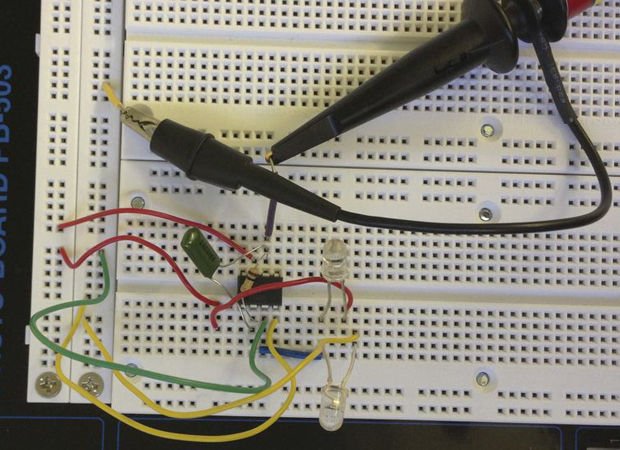Instructables user SarahandDillon made a differential light meter with a TL082 op amp; it compares the readings of two photo sensors.This works in the exact same way as the improved transimpedance amplifier, except that the current flowing out of Vout, through the resistor/capacitor barrier, to V- is now the sum of the two photodiode currents. Furthermore, the photodiode currents are flowing in different directions, since one is reverse biased with -15V and the other is reverse biased with +15 volts (the electrons are repelled away from the -15V and attracted towards the +15V).

If the -15V diode’s current is Iminus(t) and the +15V diode’s current is Iplus(t), then the total (positive) current flowing out of Vout through the barrier is Iminus(t) – Iplus(t). So Vout is now measuring the difference between the two currents, and thus it’s measuring the difference between the intensity of light into one diode versus the other!

If you bend the two diodes so that they point in opposite directions, you now have a device that measure the difference between the intensity of light on one side versus the intensity of light on the other. A differential light meter.